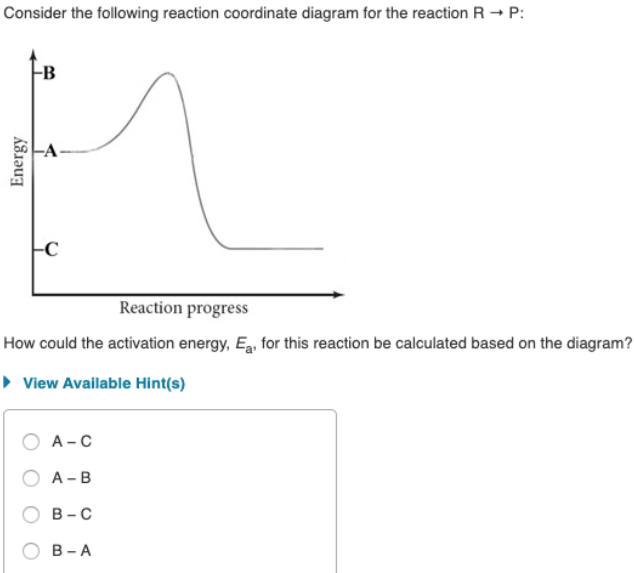# Consider the following reaction coordinate diagram for the reaction R → P: How could the activation energy, Ea, for this reaction to be calculated based on the diagram? a) A-C b) A-B c) B-C d) B-A